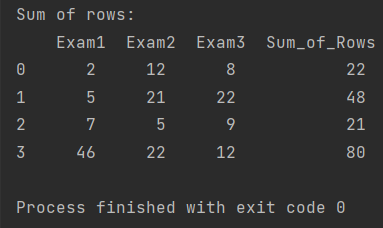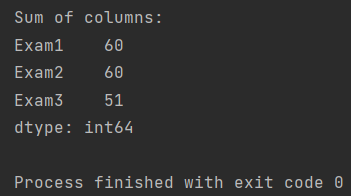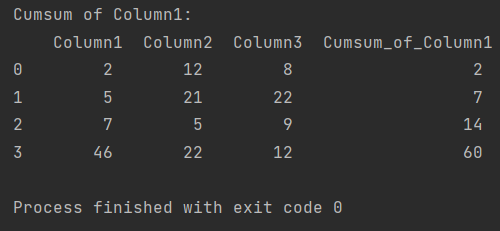Pandas How To Uncategorized How to calculate sum of rows and columns in Pandas

# How to calculate sum of rows and columns in Pandas

Here’s how to calculate sum of rows and columns in Pandas dataframe.

In this post, I will show you how to easily prepare a summary of columns and rows in a dataframe.

## How to calculate sum of rows

You can simply use the sum function to calculate the sum of the rows. As a parameter, add only axis=1 to indicate that you want to sum the rows.

```import pandas as pd

my_df = pd.DataFrame({'Exam1': [2, 5, 7, 46],
'Exam2': [12, 21, 5, 22],
'Exam3': [8, 22, 9, 12]})

my_df['Sum_of_Rows'] = my_df.sum(axis=1)
print(f'Sum of rows: \n {my_df}')

```

The summary is displayed in a separate column that I created.## How to calculate sum of columns

Also use the sum function to calculate the sum of the columns. As a parameter, add axis=0, which indicates that Pandas is supposed to sum the columns.

```import pandas as pd

my_df = pd.DataFrame({'Exam1': [2, 5, 7, 46],
'Exam2': [12, 21, 5, 22],
'Exam3': [8, 22, 9, 12]})

sum_of_columns = my_df.sum(axis=0)
print(f'Sum of columns: \n{sum_of_columns}')

```

Pandas displays a summary of the columns along with the data type they contain.## How to calculate cumsum of column

It is also possible to calculate a cumulative sum for a column in the dataframe. To do this, create a new column and indicate for which column you want to calculate the cumulative total.

```import pandas as pd

my_df = pd.DataFrame({'Column1': [2, 5, 7, 46],
'Column2': [12, 21, 5, 22],
'Column3': [8, 22, 9, 12]})

my_df['Cumsum_of_Column1'] = my_df['Column1'].cumsum()
print(f'Cumsum of Column1: \n {my_df}')

```Tags: ,

## How to join two dataframes on 2 columnsHow to join two dataframes on 2 columns

In this post I show how to join two dataframes on 2 columns in Pandas Python library.

## How to pivot a dataframe in Pandas?How to pivot a dataframe in Pandas?

In Pandas, you can use the pivot function to pivot a dataframe. The pivot function reshapes a dataframe by converting its rows into columns or columns into rows based on